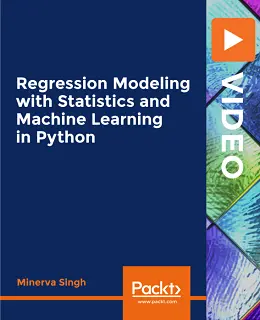# Regression Modeling with Statistics and Machine Learning in Python [Video]### Regression Modeling with Statistics and Machine Learning in Python [Video]

##### English | MP4 | AVC 1280×720 | AAC 44KHz 2ch | 6h 08m | 811 MB eLearning | Skill level: All Levels

Regression Modeling with Statistics and Machine Learning in Python [Video]: This hands-on, regression-analysis bootcamp will help you master practical statistical modeling and machine learning in Python

This course is your one-shot guide to statistical and machine learning analysis. This course will teach you regression analysis (for both statistical data analysis and machine learning) in Python – all in a practical, hands-on way.

Specifically, the course will:

• Take you from a basic level of statistical knowledge to a level where you can perform some of the most common advanced regression analysis-based techniques
• Equip you to use Python to perform various statistical and machine learning data analysis tasks
• Introduce you in a hands-on way to some of the most important statistical and machine learning concepts, so you can apply them to practical data analysis and interpretation

You will get a strong background in some of the most important statistical and machine learning concepts and their applications in regression analysis. You will be able to decide which regression analysis techniques are best suited for answering your research questions and most applicable to your data; then you’ll interpret the results.

• Harness the power of Anaconda/iPython for practical data science
• Read in data into the Python environment from different sources
• Implement classical statistical regression modeling techniques such as linear regression in Python
• Implement machine learning-based regression modeling techniques such as Random Forests and kNN for predictive modeling
• Master neural network- and deep learning-based regression

This is a practical, hands-on course we spend time dealing with some theoretical concepts related to both statistical and machine learning regression analysis.